The UCM Procedure

BLOCKSEASON Statement

• BLOCKSEASON NBLOCKS = integer BLOCKSIZE = integer <options> ;

The BLOCKSEASON or BLOCKSEASONAL statement is used to specify a seasonal component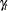that has a special block structure. The seasonalis called a block seasonal of block size m and number of blocks k if its season length, s, can be factored as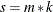and its seasonal effects have a block form—that is, the first m seasonal effects are all equal to some number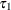, the next m effects are all equal to some number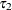, and so on.

This type of seasonal structure can be appropriate in some cases; for example, consider a series that is recorded on an hourly basis. Further assume that, in this particular case, the hour-of-the-day effect and the day-of-the-week effect are additive. In this situation the hour-of-the-week seasonality, having a season length of 168, can be modeled as a sum of two components. The hour-of-the-day effect is modeled using a simple seasonal of season length 24, while the day-of-the-week is modeled as a block seasonal component that has the days of the week as blocks. This day-of-the-week block seasonal component has seven blocks, each of size 24.

A block seasonal specification requires, at the minimum, the block size m and the number of blocks in the seasonal k. These are specified using the BLOCKSIZE= and NBLOCKS= option, respectively. In addition, you might need to specify the position of the first observation of the series by using the OFFSET= option if it is not at the beginning of one of the blocks. In the example just considered, this corresponds to a situation where the first series measurement is not at the start of the day. Suppose that the first measurement of the series corresponds to the hour between 6:00 and 7:00 a.m., which is the seventh hour within that day or at the seventh position within that block. This is specified as OFFSET=7.

The other options in this statement are very similar to the options in the SEASON statement; for example, a block seasonal can also be of one of the two types, DUMMY and TRIG. There can be more than one block seasonal component in the model, each specified using a separate BLOCKSEASON statement. No two block seasonals in the model can have the same NBLOCKS= and BLOCKSIZE= specifications. The following example illustrates the use of the BLOCKSEASON statement to specify the additive, hour-of-the-week seasonal model:

      season length=24 type=trig;
blockseason nblocks=7 blocksize=24;

BLOCKSIZE=integer

specifies the block size, m. This is a required option in this statement. The block size can be any integer larger than or equal to two. Typical examples of block sizes are 24, corresponding to the hours of the day when a day is being used as a block in hourly data, or 60, corresponding to the minutes in an hour when an hour is being used as a block in data recorded by minutes, etc.

NBLOCKS=integer

specifies the number of blocks, k. This is a required option in this statement. The number of blocks can be any integer greater than or equal to two.

NOEST

fixes the value of the disturbance variance parameter to the value specified in the VARIANCE= option.

OFFSET=integer

specifies the position of the first measurement within the block, if the first measurement is not at the start of a block. The OFFSET= value must be between one and the block size. The default value is one. The first measurement refers to the start of the estimation span and the forecast span. If these spans differ, their starting measurements must be separated by an integer multiple of the block size.

PLOT=FILTER
PLOT=SMOOTH
PLOT=F_ANNUAL
PLOT=S_ANNUAL
PLOT=( <plot request> …<plot request> )

requests plots of the season component. When you specify only one plot request, you can omit the parentheses around the plot request. You can use the FILTER and SMOOTH options to plot the filtered and smoothed estimates of the season component. You can use the F_ANNUAL and S_ANNUAL options to get the plots of "annual" variation in the filtered and smoothed estimates of. The annual plots are useful to see the change in the contribution of a particular month over the span of years. Here "month" and "year" are generic terms that change appropriately with the interval type being used to label the observations and the season length. For example, for monthly data with a season length of 12, the usual meaning applies, while for daily data with a season length of 7, the days of the week serve as months and the weeks serve as years. The first period in each block is plotted over the years.

PRINT=FILTER
PRINT=SMOOTH
PRINT=( <FILTER> <SMOOTH> )

requests the printing of the filtered or smoothed estimate of the block seasonal component.

TYPE=DUMMY | TRIG

specifies the type of the block seasonal component. The default type is DUMMY.

VARIANCE=value

specifies an initial value for the disturbance variance,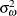, in theequation at the start of the parameter estimation process. Any nonnegative value, including zero, is an acceptable starting value.